top of page
Search
•Rafia Shabbir

# Geometric Sequence

Updated: Oct 31, 2020

A list of numbers arranged in a specific order is called as sequence of numbers.

Geometric sequence is a series of numbers in which each subsequent term is found by multiplying the previous term by a constant. This constant can be positive or negative, but it can never be zero. This constant is known as common ratio.

A geometric sequence is also known as geometric progression. In general form, we write geometric sequences like this:• a is the scale factor which means that it is the starting value of the sequence. We can also call it as the common factor of the series.

• r is the common ratio of the sequence

Remember in arithmetic sequences the numbers are arranged in such a way that the common difference between any two consecutive terms is the same. As the name implies, the common difference is found by subtracting the preceding term from the subsequent term. Arithmetic sequence is also known as arithmetic progression.

The primary difference between arithmetic and geometric sequences lies in the way they are arranged or their subsequent terms are found. These two progressions are quite common in college algebra. Once students are familiar with these two progressions, they can better understand complex Fibonacci numbers and harmonic series.

## Finding Common Ratio

Example 1

What is the common ratio of the geometric sequence { 4, 8, 16, 32, …..}.

Solution

To find the common ratio, any term in the series is divided by the preceding term.

If we take the second term 8 and divide it by the first number 4, we get the answer 2.

Similarly, when the third term 16 is divided by the second number 8 or the fourth term 32 is divided by 16, we get the same answer 2.

Hence, 2 is the common ratio.

Use the following general notation to write the series as the product of exponent and common ratio: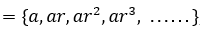= {4 , 8 , 16 , 32 , .....}

Let us evaluate another geometric sequence below:

Example 2

{3 , -9 , 27 , -81 , .....}

Solution

The above sequence is geometric because when we divide a term by the last term in the sequence, we get the constant -3. This constant -3 is the common ratio, r of this geometric series.

Note that when a common ratio is a negative number in a geometric sequence, we get an alternating sequence like above. It is also known as alternating series because the signs of the terms are alternating.

## Finding the nth term in the geometric sequence

Now, that we know what geometric sequences are and how to recognize them using a common ratio “r”. Next, we will learn how to find the nth term in the geometric progression.

The explicit formula to find the nth term of a geometric sequence is:Here, a = initial term of the sequence

r = common ratio of the progression

The above rule of geometric sequence follows a recursive relation and can be written recursively as:Here, n is an integer which is greater than or equal to 1.

Example 1

Find 13th term of the given sequence {5 , 15 , 45 , ....}.

Solution

First, determine whether this sequence is geometric or arithmetic. Since the common ratio between the consecutive numbers in the series is constant, i.e. 3, so the above numbers are arranged in a geometric sequence.

In the above question, a = 5, r = 3 and n = 8. Use the formula for finding the nth term to get the 8th term of the sequence: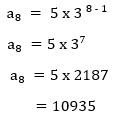The geometric sequence can also decrease and have smaller values as it progresses. Consider another example below:

Example 2

Find the 10th term of the following geometric sequence:

{9, 3.6 ,1.44 , .....}

Solution

If we divide 3.6 by 9 or 1.44 by 3.6, we get the common ratio, r = 0.4. Substituting the values of a = 9 , r = 0.4 and n = 10 in the formula, we will get the 10th term of the sequence: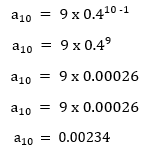Hence, the sequence gets smaller and smaller as it proceeds.

## Finding Sum of the Finite Geometric Series

The sum formula for finite geometric sequence is denoted below in summation notation:The symbol used in the above formula is known as sigma. Hence, the summation notation is also known as sigma notation.

Remember that the sum of the finite and infinite geometric series are calculated using different formulae. You may be wondering how do I find whether the question is about finite or infinite sequence. Well, here's the clue. When number of terms in a sequence for sum are specified in the question, then we will use formula of finite sequence. When they are not specified, then we assume that we need to find the sum of the series to infinity. For example,

"Find sum of first 100 natural numbers" is an example of finite sequence. On the other hand, "Find sum of natural numbers" is an example of infinite sequence.

Example 1

Find the sum of the first four terms of the following sequence:

{58 , 116 , 232 , ....}

Solution

In the question, we are asked to find the sum of the first four terms of a sequence. We don’t need to find the fourth term to find the sum of the above sequence.

In this sequence, a is the first term, r is the common ratio found by dividing the subsequent term with preceding term, for example 116/58 = 232/116 = 2. Finally, n is the number of terms for which we have to find the sum.

Put the following values in the summation formula: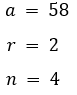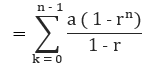Example 2

Find the sum of first 10 terms of the following geometric series:Solution

To get the sum, first, we need to find the common ratio, r of the above geometric progression.

When we divide any term by the previous term in the series, we get a constant number 1/3. Hence, this is the common ratio of the number sequence.

Plugging the values of a = 3 and r = 1/3 in the formula of sum, we will get the sum of the first 10 numbers of the above geometric sequence.0.999 is approximately equal to 1, so the sum is equal to 9/2.

## Sum of Infinite geometric series

An infinite series that has the common ratio is called an infinite geometric series. Use the following formula if you are asked to find the sum of an infinite geometric series: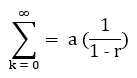The above formula has a limitation, i.e. this formula can be applied if and only if the absolute value of the common ratio |r| is less than 1.

Example 1

Find the sum of all the terms of the following geometric series:Solution

In the above sequence:Substitute these values in the following formula: